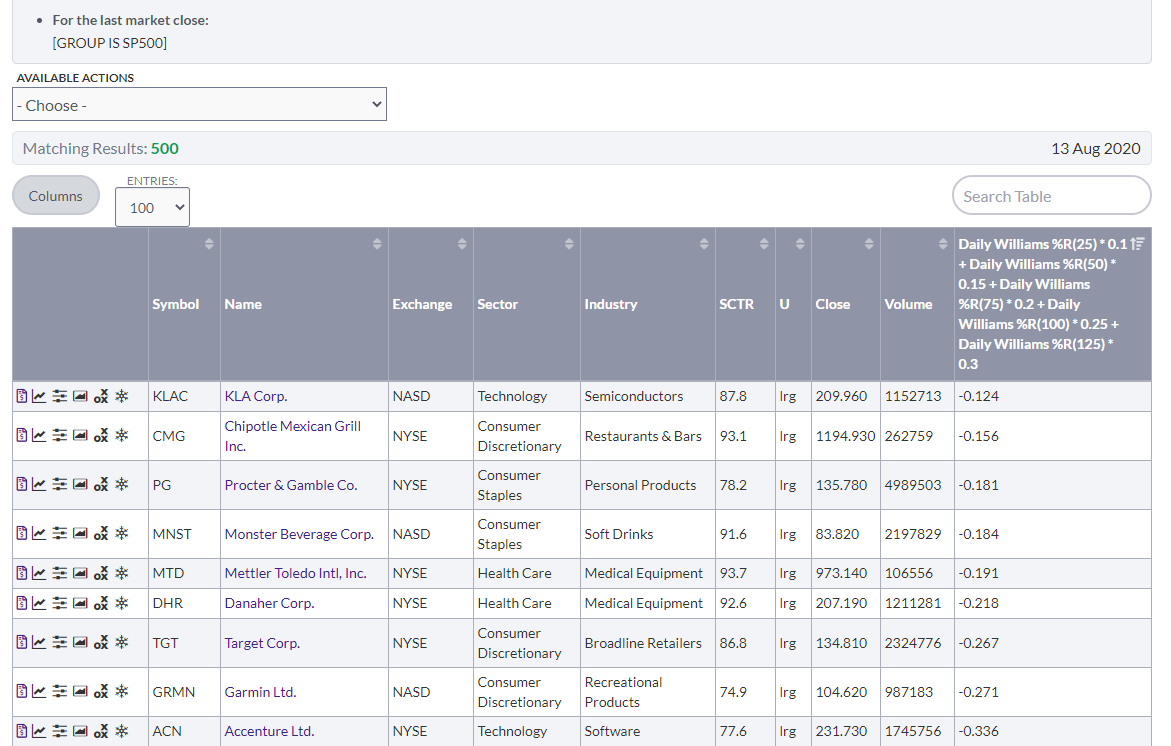#### Howdy, Stranger!

It looks like you're new here. Sign in or register to get started.

# Scan closing price / 131SMA and rank

This is a relative strength Ratio rank (the other is a momentum rank by using the ROC)
So how would you rank the stocks in group SP500 according to how farther away (Up) they Are from their 131 day SMA?
Logic is : divide close by SMA and then rank

• As far as I know, you cannot use the rank by statement with custom arithmetic operations. It accepts only built in indicators or values (e.g. MACD, yield).

You might be able to get the equivalent with the PPO indicator, which is like the MACD indicator except it expresses the result (the difference between two *E*MAs, not *S*MAs) as a per cent, which allows you to compare results between issues (which you can't do with MACD).

PPO expresses the difference between Line (which is the difference between two emas) and Signal, not the two EMAs. But, if you use parameters 1,131, 1, you effectively get the difference between close and the ema. The first parameter, 1, is the ema of the close because if the ema is 1, the ema is equal to the close. The second parameter is your 131 average - but again, the ema, not the sma. And the third parameter is the smoothing factor for difference between the first two emas (Line). But again, if the parameter is 1, the Signal is indentical to Line.

So you would write

rank by PPO Line(1,131,1)

• You can use math in the Rank By to a degree. For example, Arthur Hill shared a "weighted" Rank by where he took an indicator and weighted it by applying different values to various lengths of an indicator. I mentioned it in the link below.

https://scan.stockcharts.com/discussion/comment/6050#Comment_6050

@markd s suggested method is a very simple and clean way to achieve the desired results.
• I ran this scan, which gets no syntax errors, and got results:

[group is sp500]

rank by [Williams %R(25) *0.10 + Williams %R(50) *0.15 + Williams %R(75) *0.20 + Williams %R(100) *0.25 + Williams %R(125) *0.30]

But, the result comes out in ALPHA order, which means the rank by statement was not executed.
• Checked your code and I get 500 resultsyour